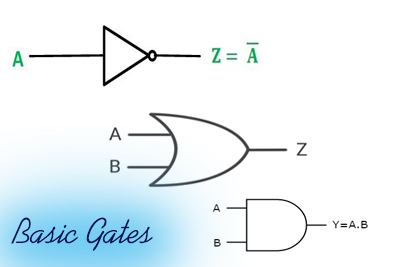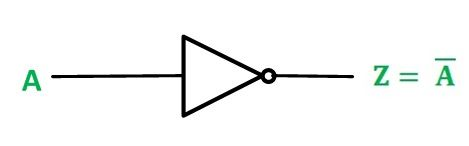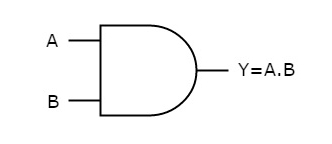Basic Gates NOT AND OR - Digital Circuits
01-03-2019    87 timesThe digital world based on the three basic gates.

• NOT Gate
• AND Gate
• OR Gate

### NOT Gate

NOT Gate is also known as inverter. This gate receives only one input and gives us just inverted signal of the input.   That is if the input is 1 then output is 0 and vise-versa.  It is symbolized asBut in the combinational circuit we can use it as a bubble. If A is the input then output symbolized as A‘ or A in digital equations.

 NOT Gate INPUT OUTPUT 1 0 0 1### AND Gate

This gate receives two or more than two inputs but gives us only one output. This circuit gives a high (1) or true output if all the applied inputs are high. An AND gate symbolized as dot (.) in digital equations. If A and B are the input then output are A.B but sometimes the dot is omitted i.e. AB.AND Gate INPUT OUTPUT A B C 0 0 0 0 1 0 1 0 0 1 1 1### OR Gate

This gate receives two or more than two inputs but gives us only one output. This circuit gives a high (1) or true output if any of the applied inputs are high. An OR gate symbolized as plus (+) in a digital equations. If A and B are the input then output are A+B.AND Gate INPUT OUTPUT A B C 0 0 0 0 1 0 1 0 0 1 1 1Query About the post